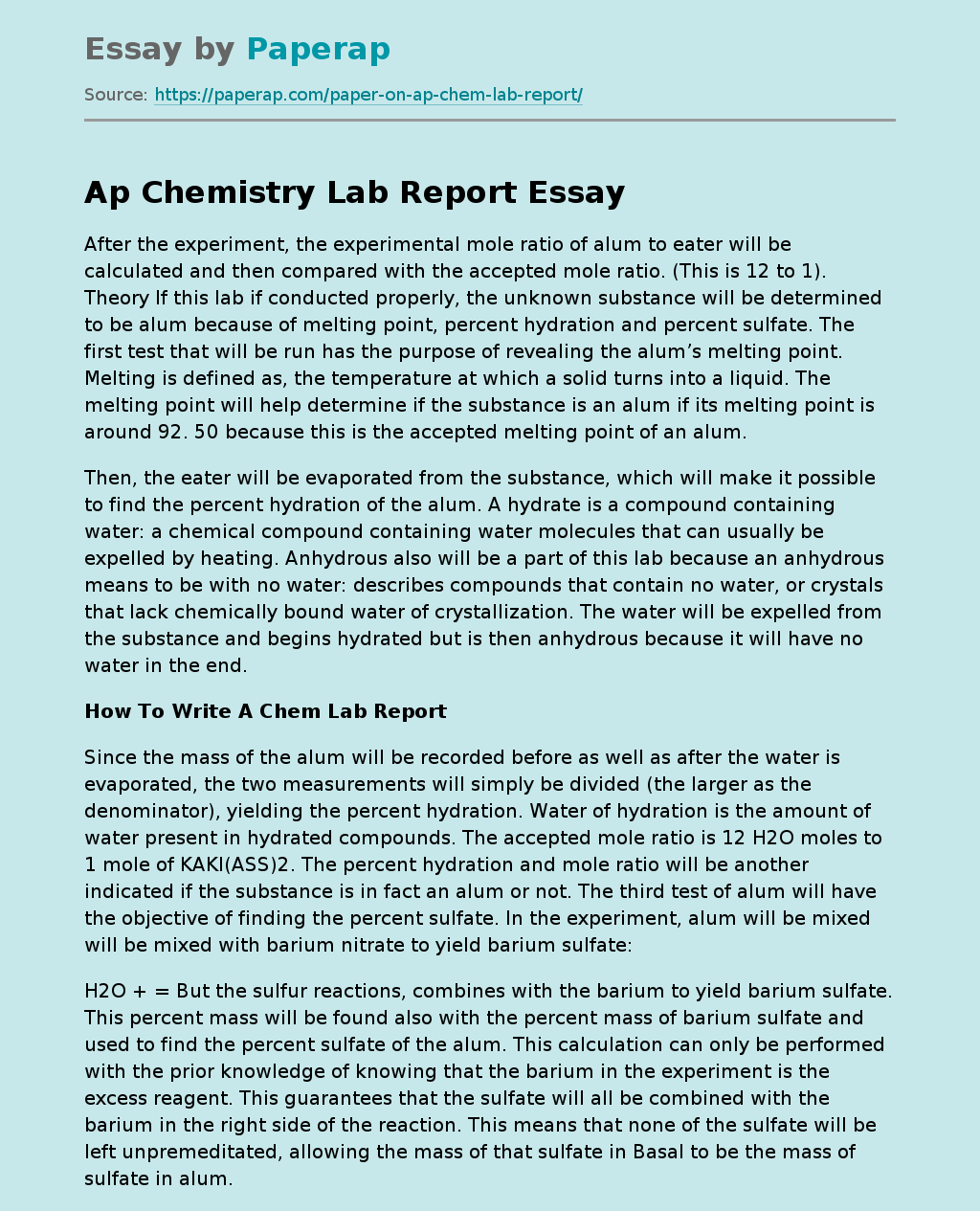# Ap Chemistry Lab Report

After the experiment, the experimental mole ratio of alum to eater will be calculated and then compared with the accepted mole ratio. (This is 12 to 1). Theory If this lab if conducted properly, the unknown substance will be determined to be alum because of melting point, percent hydration and percent sulfate. The first test that will be run has the purpose of revealing the alum’s melting point. Melting is defined as, the temperature at which a solid turns into a liquid.

The melting point will help determine if the substance is an alum if its melting point is around 92. 50 because this is the accepted melting point of an alum.

Then, the eater will be evaporated from the substance, which will make it possible to find the percent hydration of the alum. A hydrate is a compound containing water: a chemical compound containing water molecules that can usually be expelled by heating. Anhydrous also will be a part of this lab because an anhydrous means to be with no water: describes compounds that contain no water, or crystals that lack chemically bound water of crystallization.

The water will be expelled from the substance and begins hydrated but is then anhydrous because it will have no water in the end.

## How To Write A Chem Lab Report

Since the mass of the alum will be recorded before as well as after the water is evaporated, the two measurements will simply be divided (the larger as the denominator), yielding the percent hydration. Water of hydration is the amount of water present in hydrated compounds.

Get quality help nowRhizManVerified

Proficient in: Chemical Reaction4.9 (247)

“ Rhizman is absolutely amazing at what he does . I highly recommend him if you need an assignment done ”+84 relevant experts are online

The accepted mole ratio is 12 H2O moles to 1 mole of KAKI(ASS)2. The percent hydration and mole ratio will be another indicated if the substance is in fact an alum or not. The third test of alum will have the objective of finding the percent sulfate. In the experiment, alum will be mixed will be mixed with barium nitrate to yield barium sulfate:

H2O + = But the sulfur reactions, combines with the barium to yield barium sulfate. This percent mass will be found also with the percent mass of barium sulfate and used to find the percent sulfate of the alum. This calculation can only be performed with the prior knowledge of knowing that the barium in the experiment is the excess reagent. This guarantees that the sulfate will all be combined with the barium in the right side of the reaction. This means that none of the sulfate will be left unpremeditated, allowing the mass of that sulfate in Basal to be the mass of sulfate in alum.

If all of the sulfate reacts, the percent sulfate should be 40. 490. *H2O + Basal + K+ + AAA++ NON- In the above reaction, the aluminum and potassium quit their bonds with the sulfate. They abandon, so to speak, the sulfate, and become ionic substances (which explain the charges of the elements in the right side of the equation). Also, the barium abandons its bond with nitrate too, which makes the nitrate an ionic compound in the right side of the equation as well. The two substances left over, the barium and sulfate, combine to make barium sulfate.

This barium sulfate will be used to calculate the percent yield of sulfate in alum. Then, after all three of these experiments with their respective calculations are preformed, the percent error of each will be found. Procedure Melting Point: 1 . A small amount of dry alum was pulverize by a mortar and pestle. 2. The alum was packed into a capillary tube to a depth of . CM. 3. Then, to pack the alum in the capillary, the tube was bounced upside-down on the lab table. 4. The capillary tube was fastened to the thermometer. 5. The thermometer was fastened to a ring stand. 6.

The thermometer and capillary tube was then submerged into a beaker full of water and heated. As the temperature reached about 900 the heating was slowed or stopped. 7. As the first crystal melted, the temperature was recorded along with the point of all crystals melting. Determination of water hydration in alum 1. A Bunsen burner was set on a ring stand beneath a ring clamp holding a clay triangle. 2. The height of the ring stand was adjusted so that the bottom of the crucible was about LLC above the hottest part of the flame. 3. Then the crucible was heated well for about five minutes. . When the heating was completed, the crucible was cooled and massed. 5. Then about two grams of alum was put into he crucible. 6. With a lid covering most of the crucible, it was heated slowly at first, and then on high. 7. After the bubbling inside had stopped, the crucible was heated for about five minutes. 8. After the crucible cooled, it was massed. Determination of the percent sulfate 1. A filter crucible was placed in a small beaker and dried in an oven over night. 2. Using an analytical balance, about 1. G of alum was measured and placed into the dried crucible. . This was then dissolved in a ml beaker with about ml of distilled water. 4. Approximately 50. Ml of . MM Baa(NON)2 was added to the solved alum, which was then stirred until the substance was dissolved. 5. The beaker was covered and heated near boiling point for about 15 minutes. 6. The filter paper was massed. 7. The mixture was poured from above into the filtration system. 8. Once the filtering was complete, the solution was filtered again. 9. The filter paper was removed and placed in a beaker to dry overnight. 10. The beaker was massed 24 hours later.